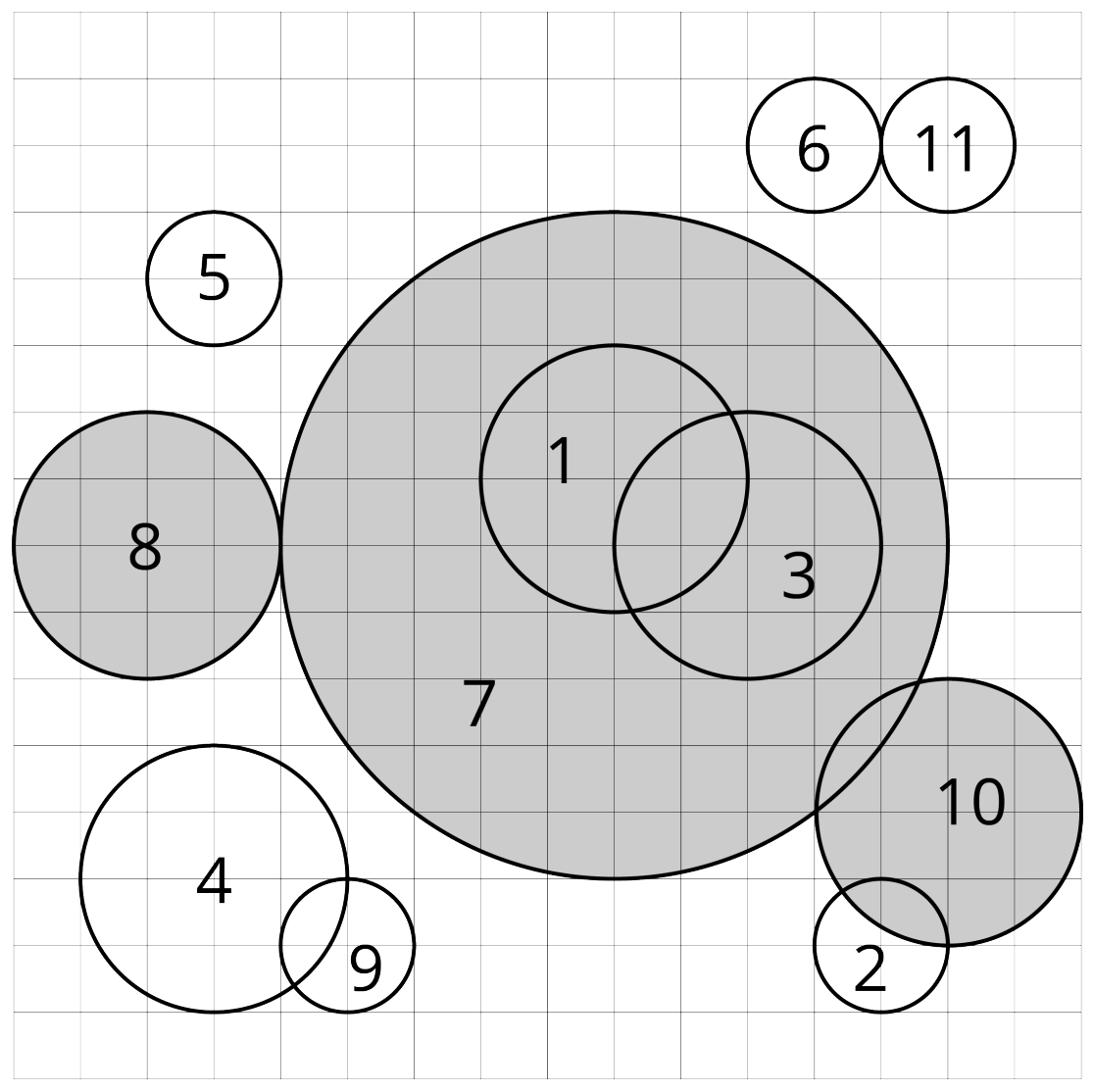시간 제한메모리 제한제출정답맞힌 사람정답 비율
3 초 1024 MB127282425.532%

## 문제

Given n circles c1, c2, . . . , cn on a flat Cartesian plane. We attempt to do the following:

1. Find the circle ci with the largest radius. If there are multiple candidates all having the same (largest) radius, choose the one with the smallest index. (i.e. minimize i).
2. Remove ci and all the circles intersecting with ci. Two circles intersect if there exists a point included by both circles. A point is included by a circle if it is located in the circle or on the border of the circle.
3. Repeat 1 and 2 until there is no circle left.We say ci is eliminated by cj if cj is the chosen circle in the iteration where ci is removed. For each circle, find out the circle by which it is eliminated.

## 입력

The first line contains an integer n, denoting the number of circles (1 ≤ n ≤ 3 · 105). Each of the next n lines contains three integers xi, yi, ri, representing the x-coordinate, the y-coordinate, and the radius of the circle ci (−109xi, yi ≤ 109, 1 ≤ ri ≤ 109).

## 출력

Output n integers a1, a2, . . . , an in the first line, where ai means that ci is eliminated by cai.

## 예제 입력 1

11
9 9 2
13 2 1
11 8 2
3 3 2
3 12 1
12 14 1
9 8 5
2 8 2
5 2 1
14 4 2
14 14 1


## 예제 출력 1

7 2 7 4 5 6 7 7 4 7 6


## 힌트

The picture in the statements illustrates the first example.